# Infinity

Infinity means beyond enumeration. Appreciation of infinity is remarkably useful mentally, emotionally, and on a personal level.

The food supply is essentially infinite in cultures where obesity is a problem; wealth is essentially infinite for those who cannot spend it all in their lifetime; and time is infinite for those who waste it. "More" in those situations is meaningless. Some scoff at primitive numbering systems that quickly reach infinity rather than using large numbers, but that is an effective way of viewing quantities larger than what is meaningful. Also, infinity reinforces the primacy of the future (which is infinite) over the past (which is merely finite).

Understanding infinity has advanced many fields, such as:

In addition, recognition of infinity — such as infinite time or infinite strength — is helpful on a personal level in dealing with adversity. Infinite time means infinite money, since time is convertible into money. Jesus often emphasized the concept of infinity in his miracles and parables (see below). He rejected the mistaken view of the ancient Greeks that infinity is non-existent and incoherent. Today acceptance of infinity is universal, despite the adamant denial by the ancient Greeks and others throughout history. The devoutly Christian mathematician Georg Cantor even proved that some infinities are larger than others.

The existence of an infinite God implies the necessary existence of the opposite: the void described in Genesis. Negative infinity, as implied by the existence of positive infinity, is the numeric equivalent of the Hell mentioned frequently in the New Testament. The logic of infinity permeates the Gospels.

## Teachings about infinity by Jesus

Infinity is depicted by several miracles, including the Multiplication of the loaves and the Miraculous catch of fish. Infinity is implicit in several parables, including the Prodigal Son, and the parable of the vineyard workers. In addition, one's soul is of infinite value to him and thus cannot be exceeded in value by anything else, as explained by Matthew 16:26.

Infinity is also portrayed by the Parables of the Hidden Treasure and the Pearl:

“The kingdom of heaven is like treasure hidden in a field. When a man found it, he hid it again, and then in his joy went and sold all he had and bought that field.
“Again, the kingdom of heaven is like a merchant looking for fine pearls. When he found one of great value, he went away and sold everything he had and bought it.”

Jesus taught the existence of infinity in multiple ways. Indeed, the Gospels and Epistle to the Hebrews are like a book of logic about infinity. This was contrary to the views of the greatest Greek mathematician and philosopher, Pythagoras and Aristotle, who both incorrectly rejected that infinity could exist. The Old Testament has only indirect references to infinity.

Eternity is infinite time, a concept that was also first introduced and emphasized by Jesus. The Old Testament has few references to "eternity" and "eternal", while the New Testament repeatedly teaches it. For example, Jesus taught about infinity in connection with Judgment Day when "these will go off to eternal punishment, but the righteous to eternal life."

## Anselm and Shakespeare

Anselm focused on infinity to prove the divinity of Christ. Chaucer referenced "infinity" in 1374, according to the Oxford English Dictionary. But Shakespeare used infinity more than anyone up until his time, in such classics as Hamlet ("A king of infinite space") and Romeo and Juliet (“My bounty is as boundless as the sea, My love as deep; the more I give to thee, The more I have, for both are infinite.”). This passage from Hamlet is particularly memorable in referring to the infinite:

 “ What a piece of work is a man! how noble in reason! how infinite in faculty! in form and moving how express and admirable! in action how like an angel! in apprehension how like a god! the beauty of the world! the paragon of animals! ”

## Similarities to zero

Infinity has surprising similarities to zero. Neither can be diminished, and the multiplication or division of either cannot change it. While infinity and zero are opposites, neither is stronger or superior to the other: multiplication of infinity by zero yields an indeterminate result. As a pair they illustrate the logical truth that "the last shall be first, and the first shall be last" as taught by Jesus at the end of the parable of the vineyard workers, a parable that illustrates that infinity cannot be diminished.

Leonhard Euler, the most prolific mathematician in history, famously said about infinity and zero:

 “ To those who ask what the infinitely small quantity in mathematics is, we answer that it is actually zero. Hence there are not so many mysteries hidden in this concept as they are usually believed to be. ”

## Infinitesimal

Inherent in the concept of infinity is the concept of infinitesimal: infinity small. Archimedes used small numbers and areas, as in calculating the area under a parabola, but the Ancient Greeks rejected the concept of infinity. Beginning with the 17th century, mathematicians like Fermat, Euler, Newton, and Leibniz used the concept of infinitesimal in integrals and differentials. Bernhard Riemann used the concept of limits to develop a rigorous definition of the integral, now known as the Riemann Integral.

## Infinity and economics

The opposite of infinity is scarcity, which is the focus of economics.

## Infinity and sports

In baseball, a pitcher has an infinite Earned Run Average (ERA) when he allows earned runs without obtaining any "outs" by the other side, because the ERA is a pitcher's average earned runs allowed per nine innings pitched (i.e., 27 outs obtained). In 2019, Nationals relief pitcher Trevor Rosenthal had an infinite ERA until he finally obtained an out.

In "Dispute over Infinity Divides Mathematicians," a commentator wrote that "[t]o determine the nature of infinity, mathematicians face a choice between two new logical axioms. What they decide could help shape the future of mathematical truth."

The commentator then discussed the continuum hypothesis, Georg Cantor, and a bit of the mathematical history of infinity.

## Infinity and mathematics

The existence of infinity implies the existence of its two opposites, zero and negative infinity. Zero is the amount that is infinitely small.

Infinity is denoted in mathematics by this symbol: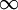, looking like an 8 on its side.

Infinity comes up in many aspects of mathematical discourse and is often treated as a number, but it is not a real number. In mathematical contexts, infinity often appears as a limit, as an integral, as the measure (size) of a set in Euclidean space, and as the cardinality (size) of sets in general.

The simplest place to see this seeming paradox is in the fact that all integers are finite, but that there are an infinite number of them.

• Why are all integers finite? Because, if infinity were an integer, what would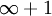be?
• Why is the set of all integers an infinite set? Because they go on forever. If the set were finite, there would be a biggest integer. But that can't be, because we can always add one to any integer. This kind of thinking is often schoolchildren's first introduction to logical reasoning.

While infinity is not a number, it appears in many other contexts. As we have seen, the size of the set of integers is infinite. We say that the cardinality of the integers (this set is often denoted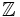) is infinite. The rational numbers () and the real numbers () also have infinite cardinality. An interesting result from set theory (see below) is thatandhave the same cardinality, whilehas larger cardinality than the other two.

Another place where infinity arises is in limits. We can say that "the limit ofas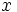approaches zero is infinity", or "the limit of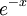asapproaches infinity is zero", but this is because infinity has a special meaning in the context of limits. See limit for discussion of this.

We can also say that "the measure (size) of the set of reals is infinity", or that certain integrals are infinite, but this is because of special properties of measures and integrals.

So infinity might arise in statements like these:

•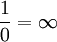Mathematics does not allow division by zero, so this is technically not allowed unless does as a limit, as follows:
•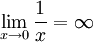Yes, one could say that "the limit is infinite," since that includes both positive and negative infinite values. But to say that the limit is "equal to infinity," one would have to say that this is the limit from the right. The limit from the left is "minus infinity," that is, unboundedly negative. This sort of statement, in terms of limits, is what was presumably meant by the incorrect statement above.
•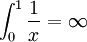Infinity has a special meaning for integrals.
•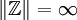The cardinality of the integers is infinite.
•The (Lebesgue) measure of the reals is infinite.

In measure theory, one sometimes defines the "extended reals", allowing plus and minus infinity to be considered to be numbers, but this is a special construction, which makes certain arithmetical operations impossible. It can't be done in general.

Another place where infinity is considered acceptable is in Floating-point arithmetic in computers. The IEEE-754 standard (which all modern computers support) allows the valuesand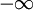. But their treatment is very different from other numbers, and computer floating-point numbers don't faithfully model the real numbers in any case.

Also, in some non-standard models of Peano Arithmetic,is treated as an actual number. But, once again, this is not standard mathematics.

Georg Cantor's diagonal argument is an elegant proof demonstrating that the (infinite) cardinality of real numbers is greater than the (infinite) cardinality of countable integers. The essence of the argument is that in any proposed list of all real numbers, a new real number not in the list can be constructed by taking the digits in a diagonal through the list and changing them to construct a new real number that differs from the nth entry at the nth position right of the decimal point.

To formalize the concepts of countably and uncountably infinite sets, we need the set theory concept of cardinality. Using this concept it can be shown that there are infinitely many distinct infinite cardinalities.

In Zermelo-Fraenkel set theory, there is the Axiom of Infinity, asserting the existence of an infinite set. The set that it creates is essentially the same as the integers. Some "constructive" mathematicians work without this axiom, and determine which results may be proved without assuming it.

## Classification of infinities

In modern mathematics, it is recognized that there is no single concept of 'infinity' - there are many different infinities. We can divide these into some broad classes. Firstly, there are the cardinals, which are used to measure the sizes of sets; and then there are ordinals, which are used to measure the position of an item in an ordered list. For finite quantities, we can use the same numbers for both - a race can have 3 participants, and you can come 3rd in the race. But, with infinite quantities, this no longer applies, the same numbers can now be used for both purposes. Thus, the smallest infinite cardinal is aleph-null, but the smallest infinite ordinal is omega.

### Cardinals

We say two sets have the same size if it is possible to put their respective members into one-to-one correspondence. A cardinal can be conceived as the equivalence class of all sets having that size.

Consider the set of all natural numbers, and its subset the set of all odd natural numbers. One would think that there are half as many odd numbers as natural numbers. Yet, while that is true when dealing with some finite subset of the natural numbers, when dealing with their entirety, it turns out that there are as many odd numbers as natural numbers. This can be shown by the fact that the natural numbers and the odd natural numbers can be put in a one-to-one correspondence: 0 -> 1, 1 -> 3, 2 -> 5, 3 -> 7, etc. (represented by the formula 2*n + 1). Thus, whereas for finite sets if A is a proper subset of B, then the cardinality of A must be less than the cardinality of B, for infinite sets this is no longer the case; an infinite set may be the same size as one of its proper subsets.

The cardinality of the natural numbers is known as aleph-null. There are as many integers as natural numbers; and as many rational numbers as natural numbers. But it can be shown, using Cantor's diagonalization argument, that the reals are strictly larger than the natural numbers - you cannot put the natural numbers into one to one correspondence with the reals. The cardinality of the reals is the cardinality of the power set of the natural numbers, which is known as the power of the continuum (c) or as beth-one. (Aleph-null is the same as beth-null; whether aleph-one is the same as beth-one is a mathematical question, which essentially has no answer - and we can even show it has no answer.)

The generalized continuum hypothesis (GCH) states that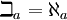for all ordinals. So, the continuum hypothesis is a special case of the generalised continuum hypothesis for ordinal. Like CH, GCH is independent of the axioms of ZFC.

A set having cardinality equal to or less than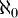is called countable; a set of greater cardinality is called uncountable.

## Ordinals

Numbers serve two distinct purposes - to measure the size of sets, and to measure the position of an item in an ordering. Thus, we may speak of a race having 2, 3, 4, 5, etc. contestants, and we can speak of the contestants as having come 1st, 2nd, 3rd etc. (or even 0th, if one is a mathematician!) When we measure the number of elements in a set, we are using cardinal numbers; when we measure an item's position in an ordering, we are using ordinal numbers. For finite quantities, it does not make that much difference, since for finite quantities we can use the same numbers to serve both purposes. But for transfinite quantities, that is no longer the case - we can no longer use the same numbers as both cardinals and ordinals. Thus, the smallest transfinite cardinal is, but the smallest transfinite ordinal is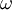.

## Other types of infinite numbers

Other than transfinite cardinals and ordinals, there are other types of infinite numbers:

• the affinely extended reals: these are elements of the set. In other words, the real numbers extended by the addition of positive and negative infinity.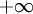is greater than every real number, andis less than every real number.
• the projectively extended reals: these are elements of the set. In other words, the real numbers extended by the addition of a single signless infinity. The ordering relation is not defined for the projectively extended reals - but informally,can be said to be both greater than and less than every real number.
• the hyperreals: denoted *, these extend the real numbers with both infinite and infinitesimal quantities. They are used in non-standard analysis. Other extensions of the real numbers with infinite and infinitesimal quantities include the surreal numbers, the superreal numbers, and the Levi-Civita field.

The conclusion to be drawn from all of this, is that although in popular usage we speak about infinity as if it were a single thing, there are actually numerous different infinities, all belonging to different systems. If we want to talk about infinity, we ought to be careful to specify which infinity we mean.

## Archimedean property

An ordered field is said to be Archimedean if it has no infinitely large or infinitely small elements. One way of stating this: an ordered field is Archimedean, if for every element of the field, there is a greater natural number. This is true for the reals - for every real number, there is a greater natural number - but not for example for the affinely extended reals, sinceis greater than every natural number. So the affinely extended reals are said to be non-Archimedean. Likewise, the hyperreal numbers, the surreal numbers, the superreal numbers and the Levi-Civita field are all non-Archimedean. Any ordered field containing infinities must also contain infinitesimals as their inverse.

To understand the notion of non-Archimedeanity, it is interesting to imagine what it would be like were space or time were non-Archimedean. If space was non-Archimedean, there could be areas of the universe infinitely distant from here. If time was non-Archimedean, there could be past times an infinite number of years before the present, and future times an infinite number of years into the future. It appears that no one has every seriously suggested that time or space actually are non-Archimedean, but it is nonetheless an interesting mental exercise to understand the notion.

## Philosophy of mathematics

Different positions in the philosophy of mathematics have different attitudes towards infinity. At one extreme, Platonists and formalists generally have no philosophical objection to any form of infinity than can be defined. At the other extreme, finitists and ultrafinitists deny the existence of infinity; to them, only finite quantities, and finite objects, actually exist. In the middle, many constructivists and intuitionists adopt a position of countablism - an acceptance of the existence of countable sets, but denying that uncountable sets exist.